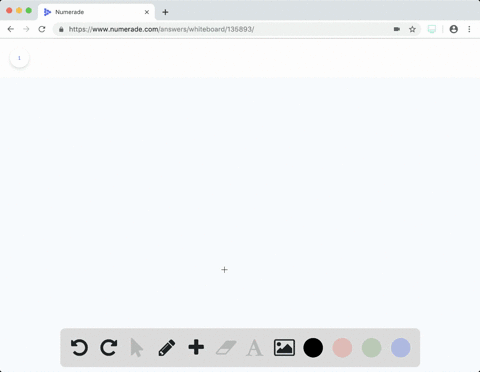### Consider the two diagrams that follow. (a) Based …

03:06Manhattan College

Need more help? Fill out this quick form to get professional live tutoring.

Get live tutoring
Problem 10

The gas-phase reaction shown, between $\mathrm{N}_{2}$ and $\mathrm{O}_{2},$ was run in an apparatus designed to maintain a constant pressure. (a) Write a balanced chemical equation for the reaction depicted and predict whether $w$ is positive, negative, or zero. (b) Using data from Appendix C, determine $\Delta H$ for the formation of one mole of the product. [Sections 5.3 and 5.7$]$

a) the distance moved is zero, which make w zero.
b) Enthalpy change for this reaction is called the enthalpy change for the formation of NO. since the
reactants are in natural states hence $\Delta H_{f}^{*}$ is equal to zero for nitrogen and oxygen.

## Discussion

You must be signed in to discuss.

## Video Transcript

Eric Guys doing problem Number ten, Chapter five of Chemistry Central Science. So the gas fees reaction been shown in your textbook between end, too. And all, too, was one run in an apparatus designed to maintain a constant pressure. So part of me wants right bounce chemical equation for the ration to pick them. Predict whether w is positive. Negative zero. So here's our balance equation and two plus. So to yields to Eno help. So now they now right about now, based on the healthy apparatus looks, it looks as if it looks as if the work being done is zero. Because although we have a constant, we have a we have a constant pressure. Remember, work is equal to W minus P. Delta V. It also looks like the volume is constant as well. There doesn't seem to be any change in the volume volume that we can see, so Delta V is equal to zero and Delta V is equal zero. That means work is equal to zero now, for Part two B, they wanted you dead. You have exceed turned Delta Asia to make information of one more of our product. So for one more of our product, but so with weak. So since then, we have two moles of our prize forms here. So we're going to rewrite this equation. One half and two plus one half o two yield an o. So remember Hess's law Delta H of reaction is equal. Delta Proxmire is dodging reactions in our death age. Reaction is going to be zero because our reactant our end to end all to these air element in their standard state. So are dealt Ege reaction is going to equal the Delta h h of our product, which is Eno. So our Delta two reaction is going to equal ninety point nine three seven heaven killer Jules.